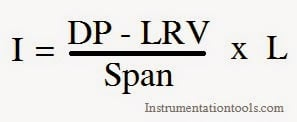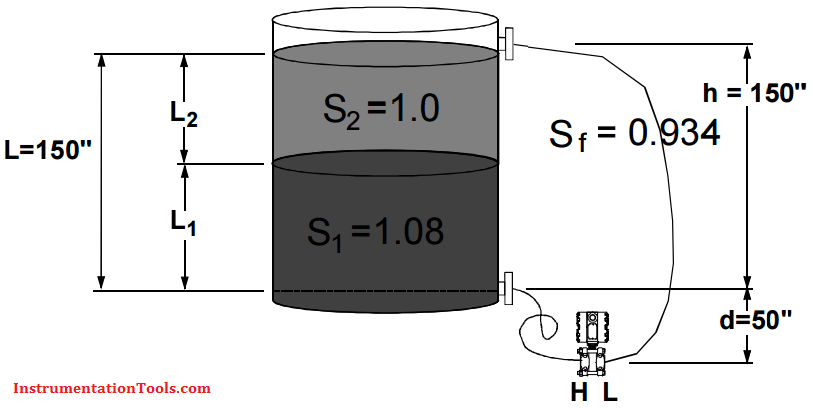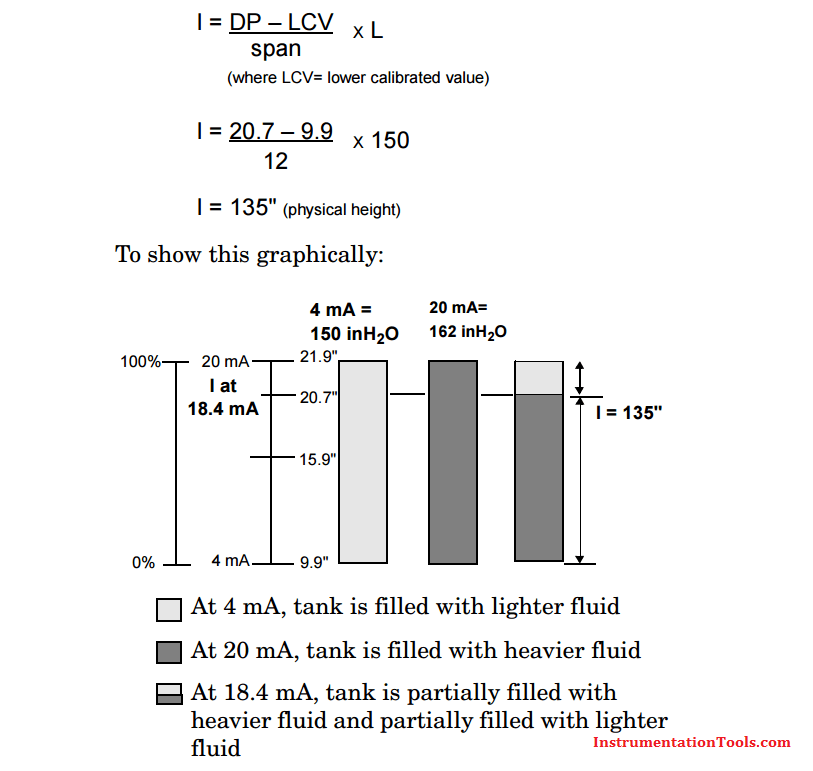# Interface Level Measurement using DP Transmitter

Differential pressure (DP) transmitters are used to measure the interface of two fluids that have different specific gravities (S1 & S2). To make an interface measurement, the overall level must be at or above the low pressure tap at all times. It is important that the level be large enough to create a reasonable DP between the two specific gravity extremes. This measurement can be done with or without remote seals.

However, from a maintenance point of view, it may be easier to use a remote seal assembly; keeping the wet leg at a constant height can be difficult in some applications.

To determine the calibrated range for the transmitter, four assumptions need to be made:

1. At the lower calibrated value, 4 mA point, the tank is filled with the lighter fluid.
2. At the upper calibrated value, 20 mA point, the tank is filled with the heavier fluid.
3. The taps leading to the transmitter are flooded at all times. The overall level should be equal to or higher than the upper (low pressure) tap.
4. There is always a reference level seen by the low pressure side. This can be accomplished with either a remote seal system or a wet leg. The reference level must have a constant height and density. The calculation is modified slightly for wet leg systems when the density of the high and low pressure wet legs differ.

The measured level is composed of a combination of the two fluids:

L = L1S1 + L2S2

When the tank is filled with the lighter fluid, the transmitter will be at 4 mA (or 0% of span) and L2 = L:

HP = L2S2 + dSf

LP = dSf + hSf

At 4 mA, DP = HP – LP = L2S2 – hSf

When the tank is filled with the heavier fluid, the transmitter will be at 20 mA (or 100% of span) and L1 = L:

HP = L1S1 + dSf

LP = dSf + hSf

At 20 mA, DP = HP – LP = LS1 – hSf

## Interface Level MeasurementCalculation for interface measurement
This tool is used to calculate the DP transmitter ranges for interface measurement application

The formulas used to calculate calibration ranges of a DP transmitter for interface measurement application

The Transmitter 4ma or LRV formula
∆Pmin or 4ma =  LS2 – HSf
The Transmitter 20ma or URV formula

∆Pmax or 20ma = LS1 – HSf

Using the above tools, we are calculated the transmitter ranges. Now calibrate the transmitter and take into line. Now note down the present DP value of transmitter for calculating the interface level.

Now enter the Span, L and LRV of transmitter in DP scale (Transmitter 4ma value in DP scale) parameters which can obtained from above interface measurement transmitter calibration tool. As we taken transmitter into line, the measured DP can be obtained. Now substitute all the parameters in the below interface calculation tool.

The formula used to calculate interface level using DP transmitter for interface measurement application are:Formula for interface level measurement
Where,
H = Distance between taps
L = Total Measured Level
S1 = Specific gravity of lighter fluid
S2 = Specific gravity of heavier fluid
Sf = Specific gravity of reference leg
Measured DP= After transmitter calibration, present DP value
Span = Difference between absolute of maximum and minimum range
LRV = Lower range value of transmitter in DP scale
I = Interface level

Interface Measurement using DP Transmitters

• Low cost
• Simple to install

Limitations

• Span must be sufficiently large enough to measure; either the distance between taps or the specific grabity difference m7ust be large
• Upper tap must be covered at all times.
• Small spans are susceptible to temperature induced errors.

Example Problem:

A vessel requires an interface measurement where the level is 150 inches. The vessel has two fluids with specific gravities of 1.0 and 1.08.At 4mA, DP = L2S2 – hSf

DP = (150 x 1.0) – (150 x 0.934)

So at 4 mA = 9.9 inH2O DP

At 20mA, DP =  LS1 – hSf

DP = (150 x 1.08) – (150 x 0.934)

So at 20 mA = 21.9 inH2O DP

The calibrated span is 9.9 to 21.9 inH20. When the transmitter reads 9.9 inH20, the tank is filled with the lighter fluid. When the transmitter reads 21.9 inH20, the tank is filled with the heavier fluid.

To determine where the interface of a mixture of fluids is, use the DP reading as a percent of span. For example, if the transmitter output is 18.4 mA or a DP of 20.7 inH2O, the interface is calculated by using the formula:Be the first to get exclusive content straight to your email.
We promise not to spam you. You can unsubscribe at any time.

### 13 thoughts on “Interface Level Measurement using DP Transmitter”

1.Really helped me…Sharing is good.

2.Hi,

•Hi, Vardhan,

Interface level measurement using radar tool will be posted soon.

Thanks,

3.Mind Blowing tools dude. Thanks for sharing these applications.

4.Could you please explain,how to calculate the range if the transmitter is installed above the HP side

Sajeesh sathyapalan

5.why you are not using d value in calculation

•Hello Ritesh, The Post is updated with detail information. Thanks for the query.

6.Regarding remote seal or wet leg, assumption no. 4 said the tube must always be filled with the liquid and the liquid should has constant density. I’m confuse, if the tube always be filled with known liquid, means that at the LP side, the pressure is constant even though no liquid in the tank, because of the liquid in the tube?

7.Hello, Yes, Even though there was no level in the tank, the Wet leg or LP side always pressure will be there and in negative number. In calibration or range calculation we compensate this extra pressure. Click Here to read the article.

8.There is an text error in your formula calculator, please change it , S2 is lighter fluid but typed heaver fluid with it in calculator.

9.Dear sir,
thanks for the sharing, I have one doubt regarding to interface level formula that Can u please explain in detail that how this formula came? then it will be very helpful for us.

10.11.